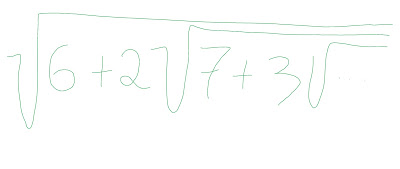## Posts

Showing posts from March, 2019

### Are there more strings than numbers?Suppose (hypothetically) that you get this question during a round of technical/math interviews: "Create a 1-1 mapping between the natural numbers and every single conceivable string" Wow. Well, you may pull up your sleeves and start thinking about it. Start randomly: 0 -> "dog" 1 -> "cat" 2 -> "IDONTKNOW" 3 -> "Infinity" ... That's not a great strategy. Clearly you need some order on the right side of the mapping. But if we think about order, you may end up in a situation where you'll convince yourself that there are way more strings than natural numbers . Without loss of generalization, we'll assume only strings in the alphabet of the uppercase English letters. Suppose that you start mapping each number to a sequence of "A"s. Take a look at image below: With this approach we have exhausted all the natural numbers (notice the Googleplex there) without even getting to reach the l

### Ramanujan Second ProblemAbout a year ago, I wrote about one of my heroes, Ramanujan, and his first really cool problem:  https://dreaktor.com/2018/02/ramanujan-first-problem.html . It turns out that he also had a less famous problem which went also unsolved for many, many years: Sorry for the handwriting paint... In any case, this is an infinite series for which I have no idea how to solve, but with the help of some simple recursive code, you can pretty much find the solution in 5min. The code will be very similar to the first post, with the exception that I'm now passing two values (the "6" as v1, and the "2" as v2) as parameters, and the number of terms I want to process. In way than less than 70 terms, the function converges fast. The answer? 4 (four) . Code and output are down below, cheers, ACC. Code: using System; using System.Collections.Generic; using System.Linq; using System.Text; using System.Threading.Tasks; namespace Ramanujan2 { class Program

### Construct Binary Search Tree from Preorder TraversalProblem is here:  https://leetcode.com/problems/construct-binary-search-tree-from-preorder-traversal/ Return the root node of a binary  search  tree that matches the given  preorder  traversal. (Recall that a binary search tree is a binary tree where for every  node , any descendant of  node.left  has a value  <   node.val , and any descendant of  node.right  has a value  >   node.val .  Also recall that a preorder traversal displays the value of the  node  first, then traverses  node.left , then traverses  node.right .) Example 1: Input: [8,5,1,7,10,12] Output: [8,5,10,1,7,null,12] Note:   1 <= preorder.length <= 100 The values of  preorder  are distinct. This is a relatively simple problem, although labeled as Medium. The definition of a binary search tree is even given in the problem description. A recursive solution does the trick: add the first node, then call recursively for the left by creating a sub-array with the smaller elements, and similar

### Palindrome Partitioning, Medium LeetcodeProblem is this one:  https://leetcode.com/problems/palindrome-partitioning/ Given a string  s , partition  s  such that every substring of the partition is a palindrome. Return all possible palindrome partitioning of  s . Example: Input:  "aab" Output: [ ["aa","b"], ["a","a","b"] ] Since the author wants all the solutions, chances are DP won't work here and a brute-force seems to be a better choice. Create a function to check whether a string is a palindrome. Then, do a brute-force recursively passing in the candidates that are palindrome. Add at the end, and return. That's it. Cheers, ACC. public class Solution { public IList > Partition(string s) { List > solution = new List >(); Partition(s, new LinkedList (), solution); return solution; } private void Partition(string s, LinkedList pal, List > solution) { if (String.IsNullOrEmpty(s)) { if (pal.Count

### Find Common CharactersThis problem was marked as "easy" on leetcode, here it is:  https://leetcode.com/problems/find-common-characters/ Given an array  A  of strings made only from lowercase letters, return a list of all characters that show up in all strings within the list  (including duplicates) .  For example, if a character occurs 3 times in all strings but not 4 times, you need to include that character three times in the final answer. You may return the answer in any order. Example 1: Input: ["bella","label","roller"] Output: ["e","l","l"] Example 2: Input: ["cool","lock","cook"] Output: ["c","o"] Note: 1 <= A.length <= 100 1 <= A[i].length <= 100 A[i][j]  is a lowercase letter This is somehow a set-problem: keep looking for the intersection of sets, where the set is the characters in each string. I use few (three) hash tables to "sto